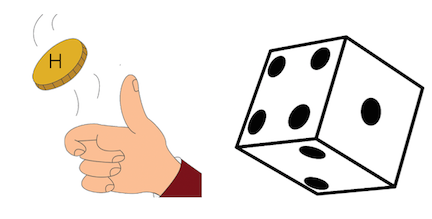# Independent Events

Probability Level 1You flip a coin and roll a die. Let $H$ be the event you flip a heads and let $F$ be the event that you roll a 4. What is $P\left(H\ | \ F\right)?$

Note: $P\left(H\ | \ F\right)$ denotes the probability of $H$ occurring given that $F$ occurs.

×